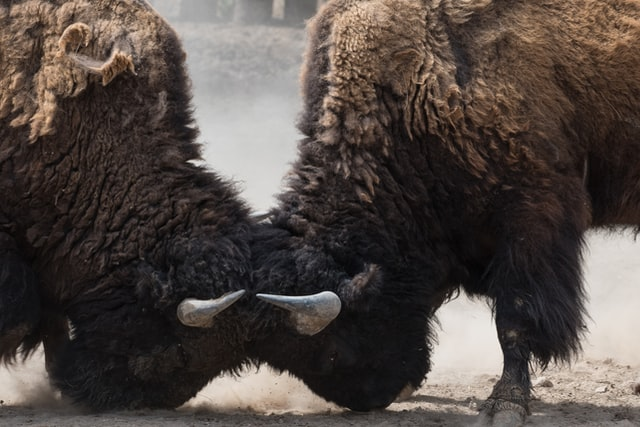# How to write a simple collision detector in HTML5 canvas and JavaScript?

Collision detection is the basics of entities interaction on canvas. Imagine you have a Player Character (PC) that’s walking through a maze and you’d like to know if this PC bumps into a wall or can keep on going. One can argue that there’s a `wall crossing potion` somewhere, but let’s assume one doesn’t have it (yet). Let’s see how we can make this work.

We won’t be starting with something as simple as a maze crawler. We are going to Help the Developer Find the Pizza. It’s a great game. You’re going to LOVE it. Oh, and we’re also going to do some… Maths…

## What is Help the Developer Find the Pizza?

In essence, there’s an image of a pizza and an image of a developer. The developer jumps randomly in the canvas looking for the pizza:

See the Pen Canvas collision detection by Yonatan Kra (@yonatankra) on CodePen.

As you can see, the developer is so wrapped up in coding, the pizza eludes them. So our coding challenge would be – create a function that finds out if the developer is on the pizza.

## How to detect collision in a Canvas?

In essence, we need to make sure that the rectangle of the developer overlaps with the rectangle of the pizza.

Let’s do a simple math lesson.

The coordinates illustrated in Figure 1 designate the leftmost X coordinate of each shape (Xd1 and Xp1) and the rightmost X coordinates (Xd2 and Xp2). `d` and `p` stand for `developer` and `pizza` respectively.

In order to know if developer and pizza collide in the X axis, we need to check the following: either our leftmost developer Xd is between the range of the Pizza’s X or our rightmost Xd is in that same range. In Math we would write it like this:

`Xp1 <= Xd1 <= Xp2 OR Xp1 <= Xd2 <= Xp2`

In code it will look like this:

`const overlapX = (Xd1 <= Xp2 && Xd1 >= Xp1) || (Xd2 <= Xp2 && Xd2 >= Xp1);`

The same applies to the Y axis. This time, the coordinates will be as shown in Figure 2.

We apply the same logic to the Y axis:

`const overlapY = (Yd1 <= Yp2 && Yd1 >= Yp1) || (Yd2 <= Yp2 && Yd2 >= Yp1);`

And we can now easily implement this in our `jump` function:

``````function jump([pizzaImage, developerImage]) {
ctx.clearRect(0,0,HEIGHT,WIDTH);

const pizza = drawStaticShape(pizzaImage);
const dev = drawJumpingShape(developerImage);

const devXRight = dev.x + dev.width;
const pizzaXRight = pizza.x + pizza.width;
const devYBottom = dev.y + dev.height;
const pizzaYBottom = pizza.y + pizza.height;

const overlapX = (dev.x <= pizzaXRight && dev.x >= pizza.x) ||
(devXRight <= pizzaXRight && devXRight >= pizza.x);
const overlapY = (dev.y <= pizzaYBottom && dev.y >= pizza.y) ||
(devYBottom <= pizzaYBottom && devYBottom >= pizza.y);
const isColliding = overlapX && overlapY;
if (isColliding) {
console.log("A Happy Developer is a Pizza-fed Developer!");
}
}``````

Notice that the drawing functions now return the coordinates and dimensions. We use them in order to calculate the right and bottom dev and pizza values (this is just like the coordinates ending with 2 like `Xd2` and `Yp2` from the equations above). We then calculate the overlap for every axis and the collision is the `AND` of both overlaps.

And now we have a rectangles collision detection algorithm we can use! You can view the full code here.

## Summary

Collision detection is one of the basics of game development. You have to know if entities collide in order to interact with each other in your game or app.

Now – I don’t want to be a party pooper, but if you’ve had enough Math for today think what would it take to calculate collision with polygons or some other custom non-uniformly curved shapes (trust me, I’ve been there and the Math is BEAUTIFUL 🙂 ).

You could, of course, avoid the Math and the whole wonderful learning process by using frameworks like phaserjs, cannojs, ammojs and more. Because my aim is to study the basics, I do not use frameworks for these kind of learning projects. Then again – one might develop a real game at some point and then one would use a framework that will make the development smoother. I do believe this hard learned knowledge of how things work under the hood comes in handy then.

Hope you enjoyed this one. As always, would love your feedback and to see what you can do with collision detection on Canvas 🙂

Featured Image Photo by Uriel Soberanes on Unsplash